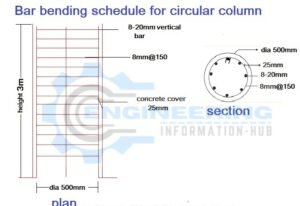# Bar Bending Schedule of Circular Column

### Bar bending schedule for circular column

what is the circular column?

the circular column is the column to use mostly for pilling and this column has used more then4  longitudinal bars of reinforcement. this column is very strong as per the square column.

now is discuss the bar bending schedule of the circular column. the bar bending schedule means the find the rebar detail in a column or any footing/foundation.detail of the rebar means the size of the rebar diameter of rebar weight of the rebar, types of rebar, and the position of rebar means how to fix the rebar in any foundation or column.

now we calculate the rebar in a circular column

# Bar Bending Schedule of Combined Footinggiven data as par sketch

diameter of column =500mm

height of column =3m

dia meter of vertical bar =20mm

dia meter of leteral  ties =8mm

concrete cover =25mm

spacing of leteral ties= 150mm

now calculation

verticals bar diameter 20mm

cutting length of vertical bar =height of column+16d(duck leg length-2bend (2d)

cutting length of vertical bar= 3000+16×20-2×20=3280=3.280m

no’s of vertical bar = 8

total length= cutting length × no’s of bar

total length= 3.280 × 8= 26.24m

weight of vertical bar = d²/162.25×total length

weight of vertical bar = 20²/162.25 × 26.24=64.69kg=65kg

leteral Ties diameter 8mm

No’s of letaral ties= height of column/spacing+1

No’s of letaral ties= 3000/150+1=20+1=21 nos

cutting length of ties = πd+(2×10dia of a bar)-(2×3dia of a bar)

so d= dia of letaral strips center to center of letaral strips

d =dia of column -2 concrete cover-dia of strips

d = 500-(2×25)-8= 500-(50)-8= 442mm

cutting length of letaral ties = πd+(2×10dia of a bar )-(2×3dia of a bar)

cutting length of letaral ties =(π×442)+(2×10×8)-(2×3×8)

cutting length of letaral ties =(π×442)+160-48=1500.58=1.500m

the total length of letaral ties  = nos of ties × cutting length of ties

the total length of letaral ties  =21×1.500=31.5m

weight of letaral ties = d²/162.25×total length

weight of letaral ties =8²/162.25×31.5=12.42kg=13kg

### Bar bendiing schedule for circular column

s.no types of bar  dia od bar (mm) no.s of bar  length (m) total length (m) weight (kg) weight (kg)
1 vertical bar 20 8 3.28 26.24 65 (w=d2/162.25xtotal lentgh
2 lateral ties 8 21 1.5 31.5 13

### Raja Numan

Hi, My name is Engr. Raja Numan author of Engineering Information Hub and I am a Civil Engineer by Profession and I've specialized in the field of Quantity Surveying, Land Surveying as QC Engineer in national and multinational companies of Pakistan & Saudi Arabia.

### Related Articles

1.gelo says:

Amazing Web-site, Keep up the useful job. Appreciate it!

슬롯사이트

2.ilove my daughter says:

3.safetoto says: